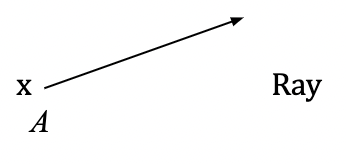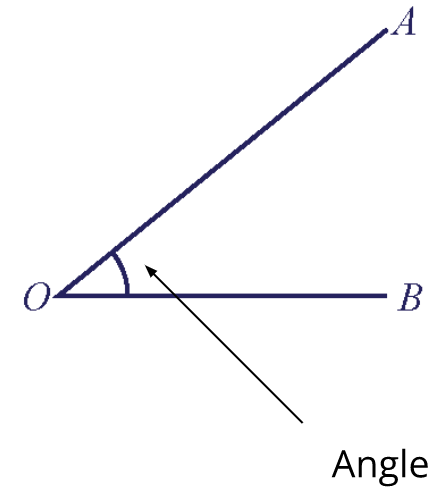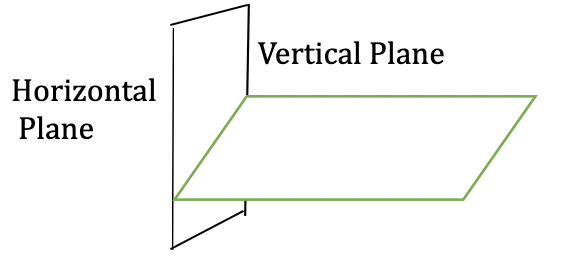Study S1 Mathematics Maths - Basic Geometry - Geniebook# Basic Geometry

In this chapter, we will be discussing the below-mentioned topics in detail:

• Basic geometrical concepts and notations
• Points, lines, planes
• Types of angles
• Complementary Vs Supplementary angles

• Properties of angles formed by intersecting lines
• Adjacent angles on a straight line
• Angles at a point
• Vertically opposite angles

## A) i) Points

 Description Representation Most basic geometrical object Connecting 2 or more points forms other geometrical objects A capital letter is used to label a point. Ex: A •     A                                              •                                                  B

## ii) Line Segments

 Description Representation Connecting 2 distinct points (called endpoints) forms a line segment A line segment, AB, is labelled by its endpoints, A and B •                                 •                   A                                B

## iii) Lines

 Description Representation A line is formed if a linesegment is extended indefinitely A line has indefinite length but no breadth or thickness x                                        x     Line      A                                       B

## iv) Rays

 Description Representation A ray is a line with only one endpoint.## v) Angles

 Description Representation An angle is formed by two rays, OA and OB, sharing the same endpoint, O. O is the vertex, while OA and OB are sides of the angle.  The angle is called angle AOB or angle BOA, written as $$\angle$$AOB or $$\angle$$BOA.## vi) Planes

 Description Representation A plane is a two-dimensional surface  A plane has length and breadth, but no thickness The floor is an example of a horizontal plane, and the wall is an example of a vertical plane.## B) Types Of Angles

 Name Definition Illustration Acute Angle $$0^\circ < x^\circ < 90^\circ$$ More than $$0^\circ$$ Less than $$90^\circ$$Right Angle $$x^\circ = 90^\circ$$Obtuse Angle $$90^\circ < x^\circ < 180^\circ$$  More than $$90^\circ$$ Less than $$180^\circ$$Straight Angle $$x^\circ = 180^\circ$$Reflex Angle $$180^\circ < x^\circ < 360^\circ$$ More than $$180^\circ$$  Less than $$360^\circ$$## C) Complementary Angles vs Supplementary Angles

 Complementary Angles Supplementary Angles Two angles are complementary if they add up to $$90^\circ$$. Two angles are supplementary if they add up to $$180^\circ$$.Let’s understand this with the help of some examples:

Question 1:

Angle POQ and angle QOR are supplementary. Angle POQ is three times the size of angle QOR. Find angle POQ.

1. $$135^\circ$$
2. $$67.5^\circ$$
3. $$22.5^\circ$$
4. $$45^\circ$$

Solution:

Let $$\angle QOR$$ be $$x^\circ$$

\begin{align*} \angle POQ &= 3x^\circ \\ \angle POQ + \angle QOR &= 180^\circ & \text { (supplementary } \angle)\\ 3x+x&=180\\ 4x&=180\\ x&=45 \\ \\ \angle POQ &=3(45)\\ &=135^\circ \end{align*}

Hence, the correct answer is Option (A).

## D) Geometric Properties Of Points And Lines

 Illustration NameCollinear Points       Three points lie on the same line.Intersecting Lines  Two lines on a plane meet at one point.Perpendicular Lines  Two lines on a plane intersect each other at right angles.Parallel Lines  Two lines on a plane do not intersect at any point.

## E) i) 1st Property Of Angles Formed By Intersecting Lines$$\begin{equation} \angle a + \angle b + \angle c =180° \end{equation}$$

 Property The sum of adjacent angles on a straight line is $$180°$$. Abbreviation adj. $$\angle s$$ on a str. line.

## ii) 2nd Property Of Angles Formed By Intersecting Lines​\begin{align} \angle a + \angle b + \angle c + \angle d = 360° ​\end{align} ​

 Property The sum of angles at a point is 360°. Abbreviation $$\angle s$$ at a pt.

## iii) 3rd Property Of Angles Formed By Intersecting Lines\begin{align*}​ \angle a &= \angle c \\ \angle b &= \angle d​ \end{align*}

 Property Vertically opposite angles are equal Abbreviation vert. opp. $$\angle s$$.

Let’s understand this with the help of some examples:

Question 2:

i) In the figure, AOB and COD are straight lines. Find the value of p.1. \begin{align*}​ p=\frac {1}{13} \end{align*}
2. \begin{align*}​ p=\frac {11}{13} \end{align*}
3. \begin{align*}​ p=11 \end{align*}
4. \begin{align*}​ p=1 \end{align*}

Solution:

\begin{align*}​ \angle AOC &= \angle DOB & \text{(vert. opp. } \angle s\text{)} \\ \\ ​ 6p+6&=7p-5\\ 6+5&=7p-6p\\ 11&=p \\ \\ \therefore\qquad p &=11 \end{align*}

Hence, the correct answer is Option (C).

ii) In the figure, AOB and COD are straight lines. Find the value of q.1. \begin{align*}​ q &=104 \end{align*}
2. \begin{align*}​ q=5\frac{3}{13} \end{align*}
3. \begin{align*}​ q=8\frac{4}{13} \end{align*}
4. \begin{align*}​ q=8 \end{align*}

Solution:

\begin{align*}\small \angle{AOC} + \small \angle{COB} &= 180^\circ & \text { (adj angles on a str. line) } \\ 6p+6+13q+4&=180^\circ\\ \end{align*}\\

Putting values of $$p=11$$

\begin{align*} 6(11)+6+13q+4&=180^\circ\\ 13q+76&=180\\ 13q&=104\\ q&=8 \end{align*}

Hence, the correct answer is Option (D).

Continue Learning
Basic Geometry Linear Equations
Number Patterns Percentage
Prime Numbers Ratio, Rate And Speed
Functions & Linear Graphs 1 Integers, Rational Numbers And Real Numbers
Basic Algebra And Algebraic Manipulation 1 Approximation And EstimationPrimaryPrimary 1Primary 2Primary 3Primary 4Primary 5Primary 6SecondarySecondary 1EnglishMaths
Basic Geometry
Linear Equations
Number Patterns
Percentage
Prime Numbers
Ratio, Rate And Speed
Functions & Linear Graphs 1
Integers, Rational Numbers And Real Numbers
Basic Algebra And Algebraic Manipulation 1
Approximation And EstimationScienceSecondary 2Secondary 3Secondary 4